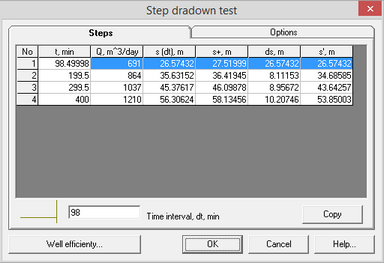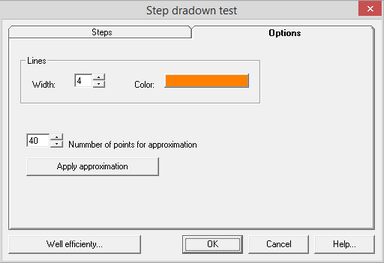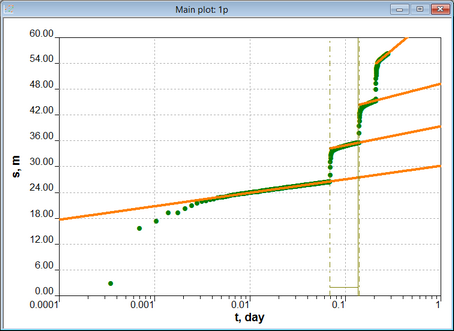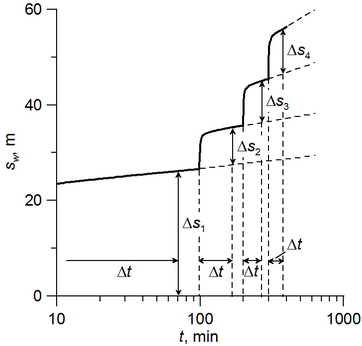﻿ ANSDIMAT+ > Aquifer tests analysis > Determination of well efficiency

# Well efficiency

Navigation:  ANSDIMAT+ > Aquifer tests analysis >

# Well efficiency

Well efficiency is normally determined with the objective to select the most suitable pumping rate for constant-rate tests allowing to achieve significant drawdown and minimise well effects at the same time. The above conditions result in aquifer tests being the most informative and successful in determination of hydraulic properties.

Well efficiency is determined by the interpretation of step-tests. A step test is an aquifer test, where pumping rate increases each step with usually 3-4 steps conducted. Pumping rates and drawdowns are recorded during the tests, and quasi steady state drawdown at the end of each step is used for interpretation.

Below is the workflow to determine well efficiency:

1) Create an ANSDIMAT project containing drawdown data and pumping rates in a pumping well for all steps;

2) Open the aquifer test project for interpretation;

3) Create plot "Qt": menu "Choose > Plot";

4) Open the dialog window of pumping rate steps: menu "Tools > Step-function";

5) In the tab "Оptions" check the box "Measurements" and press the button "Auto"; manually change steps if required;

6) press the button "OK";

7) make plot "s – lg(t)": menu "Choose > Plot";

8) Open the dialog window "Step drawdown test": menu "Tools > Step-drawdown test"; the programm will automatically approximate drawdowns on each step by straight lines and will calculate values of Δs, that are necessary for calculations of well efficiency; if required the approximating lines and time interval Δt can be manually corrected;

9) "Step drawdown test" dialog window contains all step pumping rates and calculated drawdowns that are necessary for determination of well efficiency.Data dialog window "Step drawdon test"

When the dialog window is opened the first time, the programm approximates the last measurements on each step by straight lines (Field "Number of points for approximations" in Tab "Options") using least-square-method and fills in the data dialog window based on the selected time interval (Field "Time interval" in Tab "Steps").

When required, the straight line can be moved by the mouse. When the mouse cursor is placed at the start or the end od a straight line, a circle appears. This circle can be moved by the mouse cursor on the plot and this will move the location of the straight line. Drawdowns in the table are automatically re-calculated with relocation of straight lines and changing of time interval. When a circle appears on the plot, the corresponding times are shown by vertical dash lines. The time interval of the step, that is being edited, is shown by straight vertical and horizontal lines (see the screen copy below).

Axillary buttons for moving the approximating straight lines:

Mouse + SHIFT on the start or the end point of the straight line - the line moves in parallel direction;

Mouse  + CTRL on the start or the end point of the straight line - changing the length of the line and rotation around its centre;

Mouse + ALT anywhere within the dialog window – the end of the line moves at the cursor location.

12Calculation of drawdowns for determination of well efficiency (1) and sketch illustrating calculated drawdown differences for each step (2)

Explanatory table

 Tab "Steps" Determination of drawdown differences based on measured drawdowns Table Quantity of rows in the table corresponds to a number of pumping rate steps. The table contains measured and calculated values: •Column "N" – Pumping rate step number (i = 1, 2, ...);•Column "t, min" – time at the end of the step;•Column "Q, м^3/сут" – step pumping rate;•Column "s (dt), m" – drawdown at the time Δt (see the sketch) from the beginning of the current step i;•Column "s+, m" – calculated drawdown at the time Δt from the beginning of the next step (step i + 1);•Column "ds, m" – drawdown difference (see Δs on the sketch): difference between drawdown at the time Δt step i (column "s (dt), m") and calculated drawdown for step i -1 (column "s+, m"); For the 1-step this difference equals to the drawdown at the time Δt of step 1 (column "s (dt), m");•Column "s', м" – sum of drawdown differences (column "ds, m") from the 1-st step to the i-th step  Information window for units conversion appears after pressing right mouse button within the first or the second columns of the table Field "Time interval" This is a fixed time interval (see Δt  interval on the sketch).This interval is the same for all steps and it is measured from the start of each step. This time interval is used by the programm in determination of drawdown differences. By default, when the dialog window is opened the first time, Δt is automatically assigned as the length of the shortest step period. When this interval is manually changed, drawdown differences are automatically recalculated. Pressing left mouse + CTR will open a new window, where time can be converted in different measurement units. Button "Copy" Copies the table on the clipboard Button "Well efficiency" Opens the dialog window "Well efficiency" and  copies there the table data in columns "Q, m^3/day" и "s', m".  Well efficiency is calculated by the Jacob method (Jacob, 1947). Tab "Options" Frame "Lines" Colour and thickness of approximating lines Field "Number of points for approximatioin" Determines quantity of points that are used for approximation by the least-square method. The points are counted from the end of each interval. When the quantity of points is changed, the approximating lines are automatically re-drawn for all steps Button "Apply approximation" Apply approximation by the least-square method. This button can be used in case the lines are manually moved, but a user wants to come back to the earlier approximation